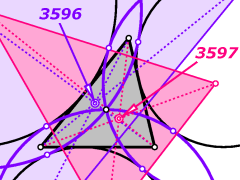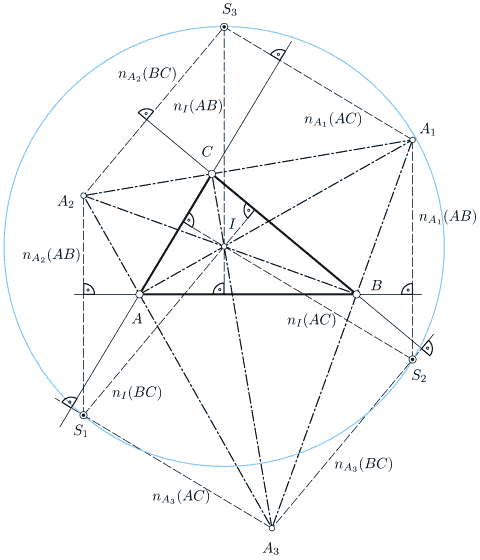# Elementary Geometry

For any researcher with a love of geometry, enticing problems remain.## The Odehnal points

B. Odehnal. Some triangle centers associated with the circles tangent to the excircles. Forum Geometricorum 10 (2010), 35-40.

Among the eight circles touching all three excircles of a triangle we find the three side lines, the Feuerbach circle, and its inverse with respect to the radical circle of the excircles.
The remaining three tritangent circles have a common point, i.e., the Spieker centre. Their contact points with those excircles which are enclosed by them form a triangle which is in perspective with the base triangle. The centre of perspectivity is the first Odehnal point X3596. The centres of these three tritangent circles form a triangle which is also in perspective with the base triangle. The centre of perspectivity is the second Odehnal point X3597.

B. Odehnal. Three points related to the incenter and excenters of a triangle. Elem. Math. 61/2 (2006), 74-80.

In the present paper it is shown that certain normals to the sides of a triangle A,B,C passing through the excentres A1, A2, A3, and the incentre I are concurrent. The triangle built by these three points (S1,S2,S3) has the incenter of A,B,C for its circumcentre. The radius of the circumcircle is twice the radius of the circumcircle of A,B,C. Some other results concerning the triangle S1, S2, S3 are stated and proved.## Publications

Hans Havlicek
Boris Odehnal
A Tribute to Rolf Riesinger (1945-2018)
Hellmuth Stachel
SitemapElementargeometrie